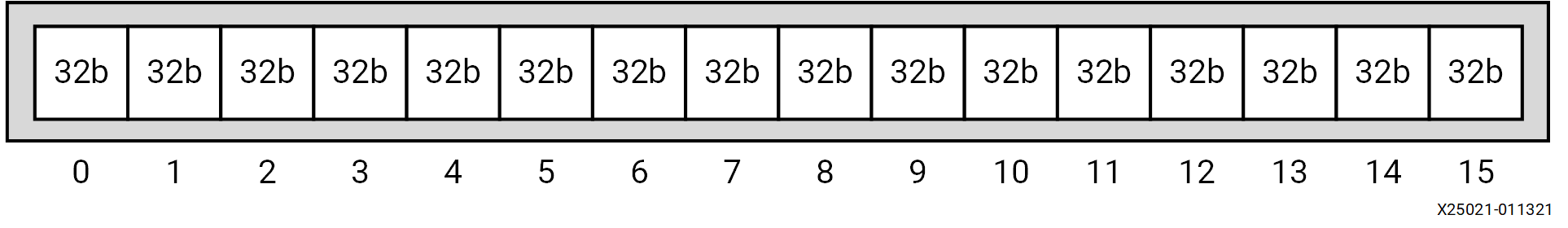# AI Engine Data Types - 2022.1 English

## AI Engine Kernel Coding Best Practices Guide (UG1079)

Document ID
UG1079
Release Date
2022-05-25
Version
2022.1 English

The AI Engine scalar unit supports signed and unsigned integers in 8, 16, and 32-bit widths, along with some single-precision floating-point for specific operations.

The AI Engine vector unit supports integers and complex integers in 8, 16, and 32-bit widths, along with real and complex single-precision floating-point numbers. It also supports accumulator vector data types, with 48 and 80-bit wide elements. Intrinsic functions such as absolute value, addition, subtraction, comparison, multiplication, and MAC operate using these vector data types. Vector data types are named using a convention that includes the number of elements, real or complex, vector type or accumulator type, and bit width as follows:

``v{NumLanes}[c]{[u]int|float|acc}{SizeofElement}``

Optional specifications include:

`NumLanes`
Denotes the number of elements in the vector which can be 2, 4, 8, 16, 32, 64, or 128.
`c`
Denotes complex data with real and imaginary parts packed together.
`int`
denotes integer vector data values.
`float`
Denotes single precision floating point values.
Note: There are no accumulator registers for floating-point vectors.
`acc`
Denotes accumulator vector data values.
`u`
Denotes unsigned. Unsigned only exists for int8 vectors.
`SizeofElement`
Denotes the size of the vector data type element.
• 1024-bit integer vector types are vectors of 8-bit, 16-bit, or 32-bit vector elements. These vectors have 16, 32, 64, or 128 lanes.
• 512-bit integer vector types are vectors of 8-bit, 16-bit, 32-bit, or 64-bit vector elements. These vectors have 4, 8, 16, 32, or 64 lanes.
• 256-bit integer vector types are vectors of 8-bit, 16-bit, 32-bit, 64-bit, or 128-bit vector elements. These vectors have 1, 2, 4, 8, 16, or 32 lanes.
• 128-bit integer vector types are vectors of 8-bit, 16-bit, or 32-bit vector elements. These vectors have 2, 4, 8, or 16 lanes.
• Accumulator data types are vectors of 80-bit or 48-bit elements These vectors have 2, 4, 8, or 16 lanes.

The total data-width of the vector data-types can be 128-bit, 256-bit, 512-bit, or 1024-bit. The total data-width of the accumulator data-types can be 320/384-bit or 640/768-bit.

For example, v16int32 is a sixteen element vector of integers with 32 bits. Each element of the vector is referred to as a lane. Using the smallest bit width necessary can improve performance by making good use of registers.

Figure 1. v16int32